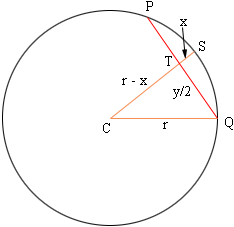Quandaries and Queries If you slice any circle with a line, and call the distance of the line between intersections the "y" length and the perpendicular length to the shorter side of the curve the "x" length, what is the resulting equation for the radius? Hi Peter, I drew a daigram of the situation you describe.The line segment PQ is of length y and the line segment ST is of length x. I let r be the radius of the circle. You know y and x and you want to find r. Since triangle TCQ is a right triangle |CQ|2 = |QT|2 + |TC|2 hence r2 = (y/2)2 + (r - x)2  Solve for r. Penny Go to Math Central